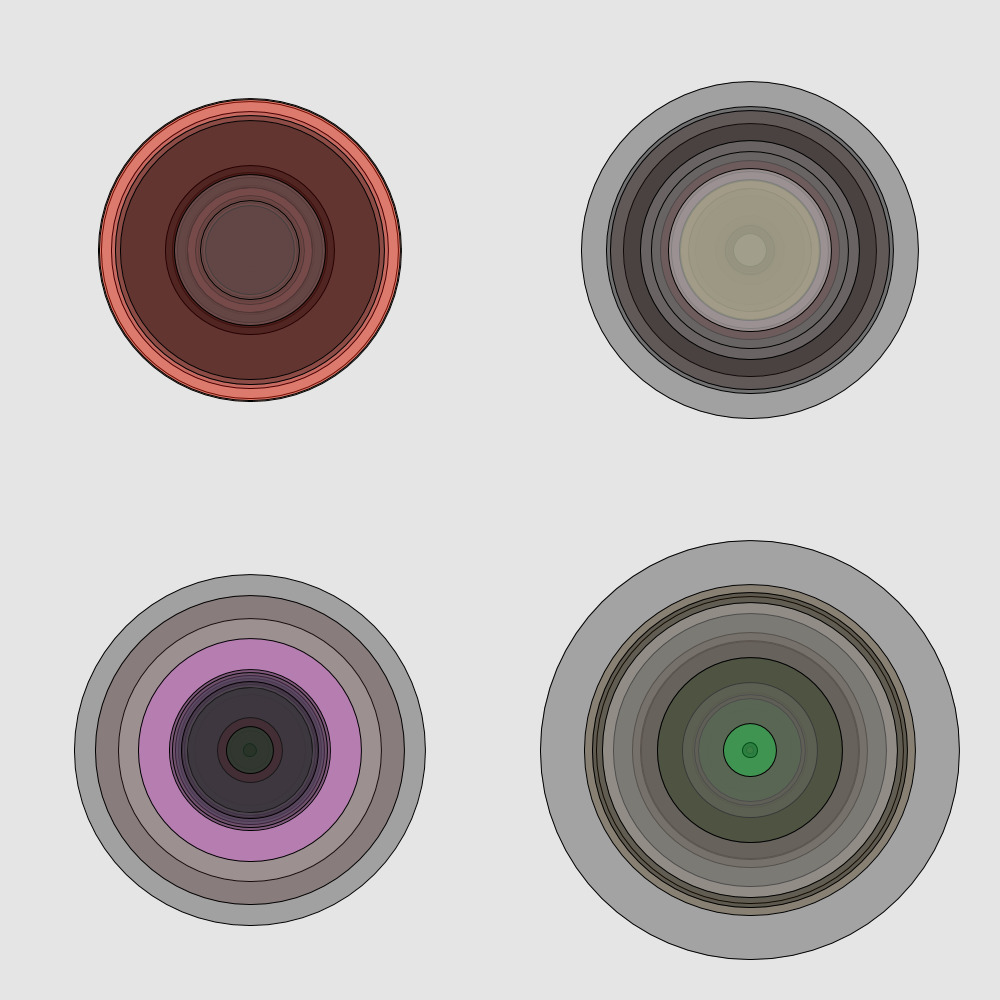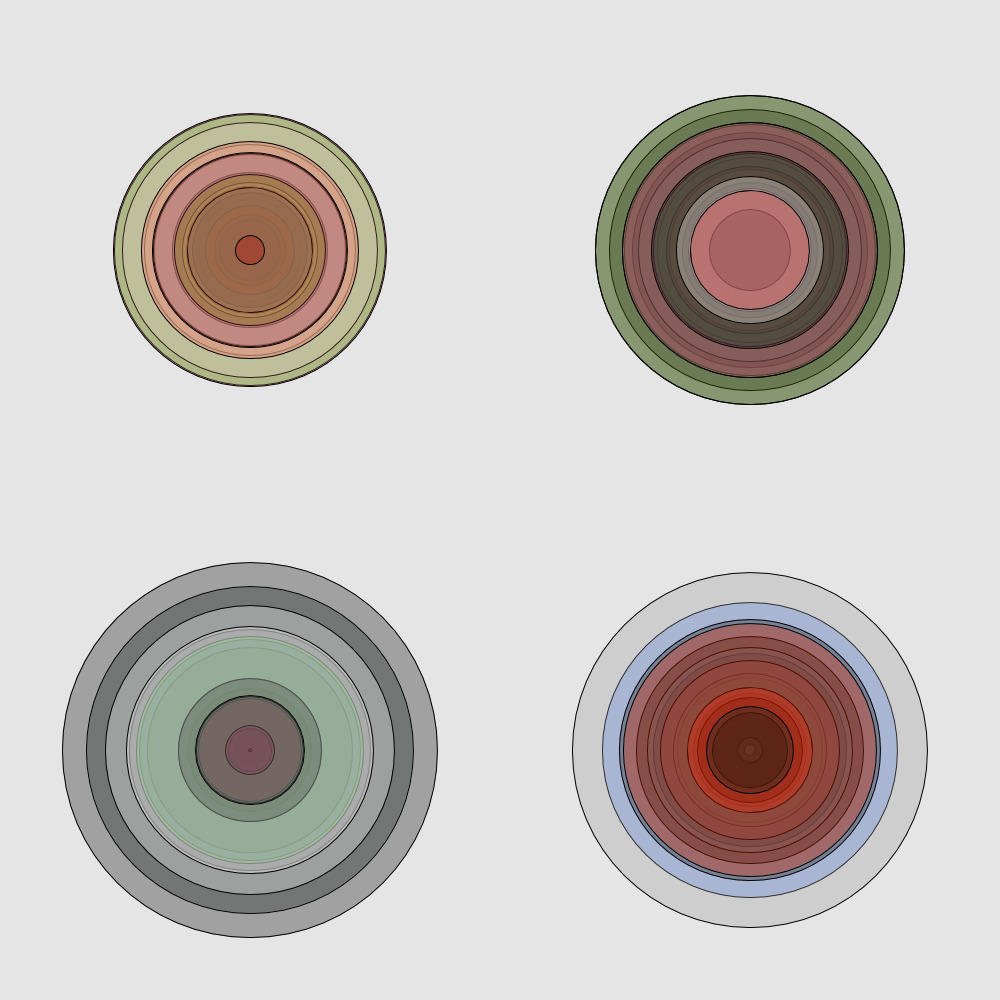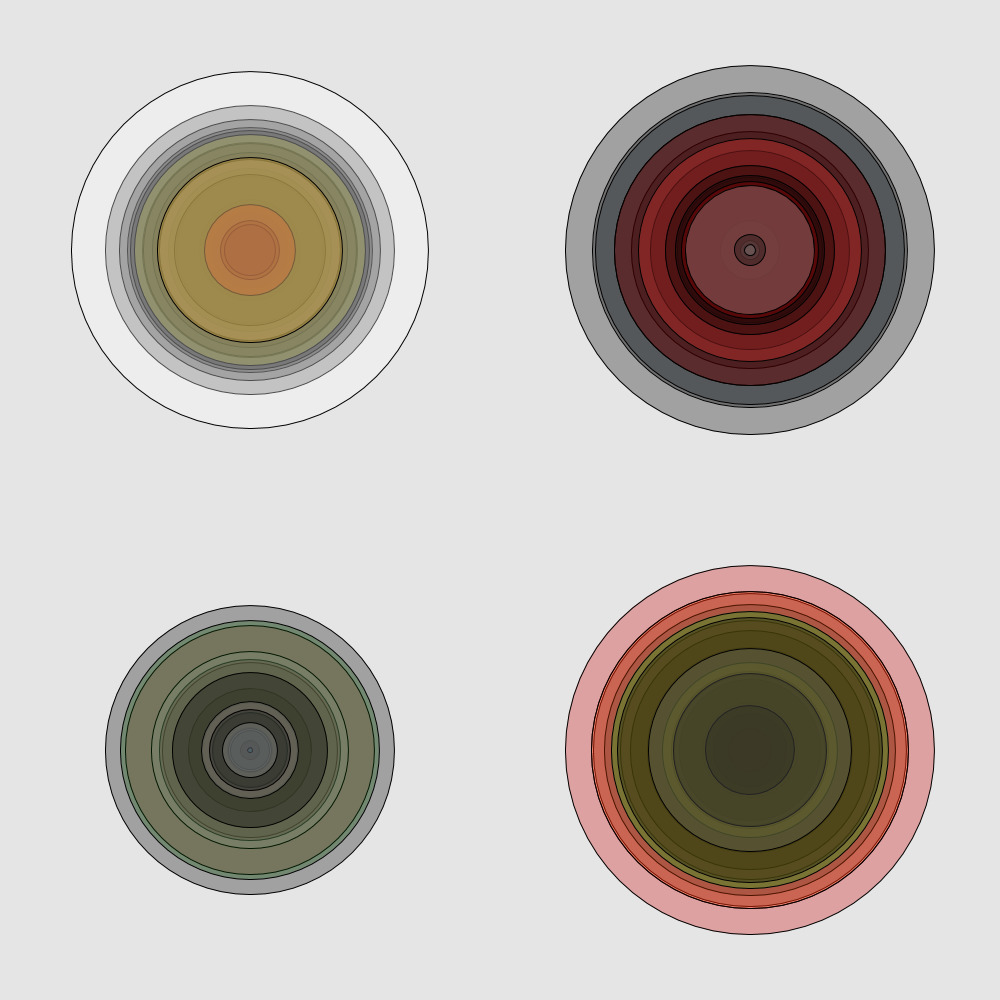# Generative Art with Quil and Processing

2018-10-13 09:59

I recently read an article on generative art. The space is incredible, and using controlled randomness to generate art seems like an amazing idea. I started looking at Georg Nees’, and found my way to Processing. The gut instinct is always to look for a Python implementation, but I was unhappy with unweildly most were. I found Quil by chance, and fell in love.

Quil weilds the Processing API in one hand, and Clojure in the other, using some fantastic language abstractions to make generating art a breeeze. I grokked it fairly easily, which resulted in the following pieces; which are simply a few concentric circles (the radii and colors of which follow gaussian distributions).Clojure code:

(ns gen_art.dynamic
(:require [quil.core :refer :all])
(:require [incanter.core :as math])
(:use [incanter.core :only [\$=]])
(:use [clojure.math.combinatorics :only [combinations cartesian-product]])
(:use [clojure.pprint])
(:use [clojure.set :only [union]])
(:use [clojure.contrib.map-utils :only [deep-merge-with]])
(:import [org.apache.commons.math3.distribution ParetoDistribution])
(:import [processing.core PShape PGraphics]))

(defn setup []
(frame-rate 10))

(defn draw []
(color-mode :hsb 360 100 100 1.0)
(background 0 0 90)

(def x 500)
(def y 500)

(defn gauss [mean variance]
(+ mean (* variance (random-gaussian))))

(doseq [i (range 20)]
(let [actual-rad (+ min-ellipse-rad (gauss 0 100))]
(fill (gauss 0 50) (gauss 50 100) (gauss 50 100) 0.3)

(doseq [i (range 20)]
(let [actual-rad (+ min-ellipse-rad (gauss 0 100))]
(fill (gauss 50 100) (gauss 50 100) (gauss 50 100) 0.3)

(doseq [i (range 20)]
(let [actual-rad (+ min-ellipse-rad (gauss 0 100))]
(fill (gauss 100 150) (gauss 50 100) (gauss 50 100) 0.3)